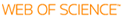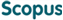### BROWSE

#### Related ResearcherKwon, Bongsuk
Partial Differential Equations and their applications
Research Interests
• Partial differential equations, hyperbolic conservation laws, stability of nonlinear waves

Maxwell solutions in media with multiple random interfaces

Cited 0 times inCited 0 times inTitle
Maxwell solutions in media with multiple random interfaces
Author
Keywords
Evolution of probability distribution; Maxwell equations; Monte Carlo simulation; Polynomial chaos; Random interface; Random media; Stochastic partial differential equation
Issue Date
2014-03
Publisher
ISCI-INST SCIENTIFIC COMPUTING & INFORMATION
Citation
INTERNATIONAL JOURNAL OF NUMERICAL ANALYSIS AND MODELING, v.11, no.1, pp.194 - 213
Abstract
A hybrid operator splitting method is developed for computations of two-dimensional transverse magnetic Maxwell equations in media with multiple random interfaces. By projecting the solutions into the random space using the polynomial chaos (PC) projection method, the deterministic and random parts of the solutions are solved separately. There are two independent stages in the algorithm: the Yee scheme with domain decomposition implemented on a staggered grid for the deterministic part and the Monte Carlo sampling in the post-processing stage. These two stages of the algorithm are subject of computational studies. A parallel implementation is proposed for which the computational cost grows linearly with the number of random interfaces. Output statistics of Maxwell solutions are obtained including means, variance and time evolution of cumulative distribution functions (CDF). The computational results are presented for several configurations of domains with random interfaces. The novelty of this article lies in using level set functions to characterize the random interfaces and, under reasonable assumptions on the random interfaces (see Figure 1), the dimensionality issue from the PC expansions is resolved (see Sections 1.1.2 and 1.2).
URI
ISSN
1705-5105
Appears in Collections:
SNS_Journal Papers
Files in This Item: